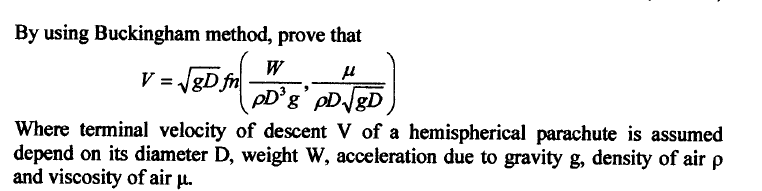# Number of repeating variable

## Homework Statement

Is the question wrong ?## The Attempt at a Solution

I found that the number of dimension = 6 , primary dimensionof velocity is L / T , which is 2 , number of repeating variable = 2 , so , there are 6-2 = 4 pi group , why the question only gt 3 pi group ?

Last edited:
•Dougias

How to do this question?

haruspex
•